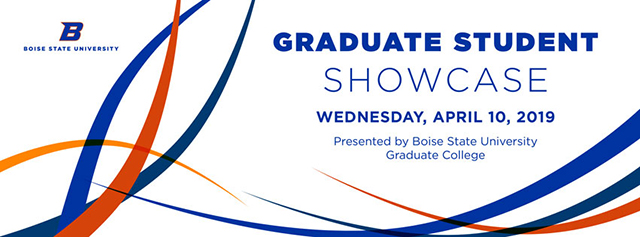#### Title of Submission

Formally Verifying the Consistency of Arithmetic

Mathematics, MS

Randall Holmes

Scholarly Poster

#### Abstract

From the very dawn of their field, mathematical logicians have historically studied the consistency of mathematics, particularly the problem of proving the consistency of Peano arithmetic (PA). In 1930, Gödel's 2nd incompleteness theorem quickly became regarded as having shown this goal impossible, yet in 1936, Gentzen’s consistency proof gave a surprising but controversial counterpoint. Our work here is concerned with implementing a version of Gentzen’s proof in Coq, an interactive theorem prover. This will show that the consistency of arithmetic is computer verified, if one accepts Gentzen's mathematical assumptions.

COinS